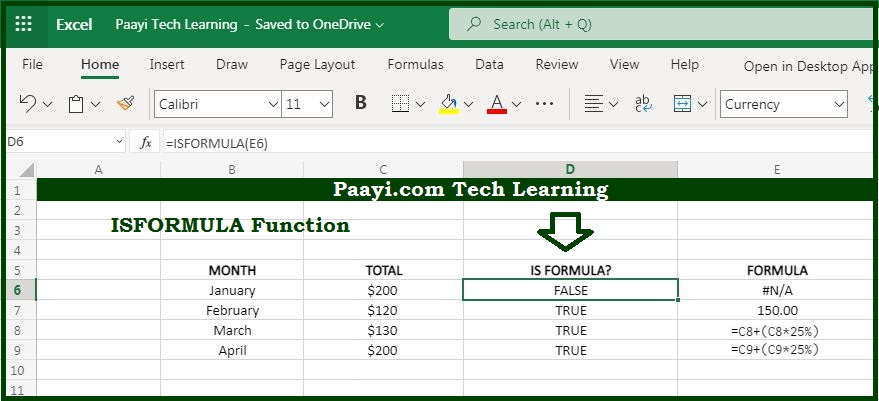# Learn How to Use Microsoft Excel ISFORMULA Function

Written by | 0 Comments | 704 Views

In this article, you will learn how to use the Microsoft Excel ISFORMULA function and its prime function in Microsoft Excel. You will also get to know the Microsoft Excel ISFORMULA function return value and syntax with the help of some examples.

Microsoft Excel ISFORMULA Function

The main function of the Microsoft Excel ISFORMULA function is to test if the cell contains a formula or not. That means with the help of the ISFORMULA function you can able to get the return value as TRUE if there is a formula in the cell and FALSE if the cell does not contain any formula. The ISFORMULA function will return TRUE regardless of the formula is wrong or the output is having an error.

Return Value of ISFORMULA Function

The return value will be TRUE or FALSE.

Syntax of ISFORMULA Function

=ISFORMULA(reference)

Where the arguments:

reference: It is the reference to a cell or cell range.

## How to Use Microsoft Excel ISFORMULA Function?You can use the ISFORMULA function to check whether the cell contains any formula or not. With the ISFORMULA function, you can able to get the return value as the TRUE if there is a formula and FALSE if there is not any formula in the cell. You can also able to display all the formulas temporarily with the keyboard shortcuts. With the FORMULATEXT function, you can able to extract and display the formulas.